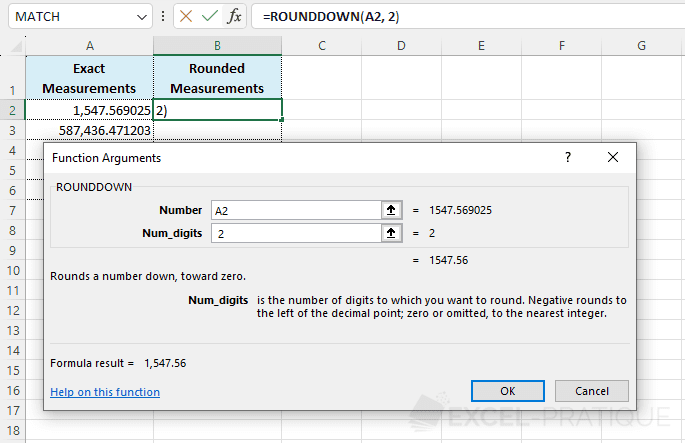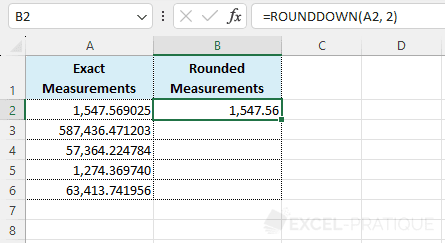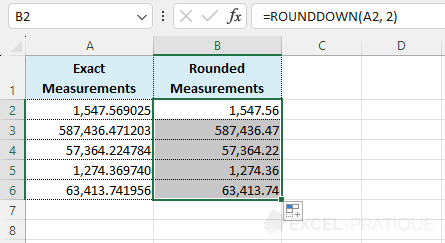# Excel Function: ROUNDDOWN

The Excel function ROUNDDOWN allows you to round a number to its lower value based on the chosen number of decimals.

Usage:

`=ROUNDDOWN(number, num_digits)`

## Usage Example

Select the ROUNDDOWN function and enter:

• Number: the number to round
• Num_digits: the desired number of decimals
``=ROUNDDOWN(A2,2)``Click OK.

The number is rounded.Then simply drag the formula down: